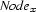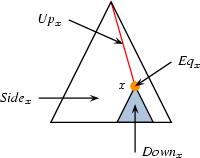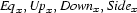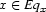### 4.3.1 Well-Formedness Constraints

Consider a solution tree for a description. Further consider the node, in that tree, interpreting variableoccurring in. When observed from the vantage point of this node, the nodes of the tree (hence the variables that they interpret) are partitioned into 4 regions:itself, all nodes above, all nodes below, and all nodes to the side (i.e. in disjoint subtrees).The variables ofare correspondingly partitioned: all variables that are also interpreted by, all variables interpreted by the nodes above, resp. below or to the side. We introduce 4 variables to denote these sets:Clearly,is one of the variables interpreted by:Furthermore, as described above, these sets must form a partition of the variables occurring in:Denys Duchier
Version 1.2.0 (20010221)